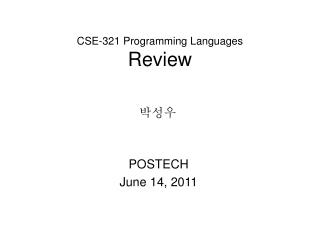DownloadDownload PresentationCSE-321 Programming Languages Review

# CSE-321 Programming Languages Review

Télécharger la présentation## CSE-321 Programming Languages Review

- - - - - - - - - - - - - - - - - - - - - - - - - - - E N D - - - - - - - - - - - - - - - - - - - - - - - - - - -
##### Presentation Transcript

1. CSE-321 Programming LanguagesReview 박성우 POSTECH June 14, 2011

2. Topics Covered • SML programming • Inductive proofs • -Calculus • Operational semantics • De Bruijn indexes • Simply-typed -calculus • Abstract machine • Subtyping, Object-orientation (Assignment 7) • Recursive type • Polymorphism • Type reconstruction, W-algorithm • Compilation (Assignment 8, Part 2)

3. 321 강의의 범위 • CMU 15-312 Principles of Programming Languages 수준 • http://www.cs.cmu.edu/~rwh/courses/ppl/ • http://www.cs.cmu.edu/~rwh/courses/ppl/schedule.html

4. Assignments • A1: 26, 95.27 (SML programming 1) • A2: 26, 92.4 (SML programming 2) • A3: 26, 81.54 (SML programming 3) • A4: 25, 89.6 (Implementing -calculus) • A5: 25, 91.4 (Typechecking) • A6: 22, 70.18 (De Bruijn indexes) • A7: 18, 79.17 (Featherweight Java) • A8: ?, ? (Type reconstruction and translation)

5. 2006 vs. 2007 vs. 2009 vs. 2010 • Assignment 4 (Implementing -calculus) • average 2006: 88.0 • average 2007: 88.57 • average 2009: 90.17 • average 2010: 93.48 • average 2011: 89.6 • Assignment 6 (De Bruijn indexes) • average 2007: 59.13 • average 2009: 56.12 • average 2010: 54.55 • average 2011: 70.18 • Assignment 7 (Featherweight Java) • average 2006: 71.30 • average 2007: 77.71 • average 2009: 68.13 • average 2010: 66.92 • average 2011: 79.17

6. 절대 평가 • Exam 20% * 2 • Quiz 10% • Assignment 50% • A1, A2, A3: 2.5% • A4: 5% • A5: 5% • A6: 10% • A7: 7.5% • A8 (Part 1): 15% • A8 part 2: Extra 25%

7. 학점

8. LICS 2011(Logic in Computer Science) http://www.postech.ac.kr/~gla/cs433/index.html

9. Paper-and-Pencil Proof

10. Proof in Coq • Strings of matched parentheses Inductive T : Set := | eps : T | cons : E -> T -> T. Inductive mparen : T -> Prop := ... Inductive lparen : T -> Prop := ... Theorem mparen2lparen : forall s:T, mparen s -> lparen s. Proof. intros s H. induction H. apply Leps. rewrite <- concat_eps. auto using Leps, Lseq. auto using lparenConcat. Qed.

11. Pythagoras' theorem • Proof Theorem even_is_even_times_even: forall (n : nat), even (n * n) -> even n. Theorem main_thm: forall (n p : nat), n * n = double (p * p) -> p = 0. Definition irrational (x : R) : Prop := forall (p : Z) (q : nat), q <> 0 -> x <> (p / q)%R. Theorem irrational_sqrt_2: irrational (sqrt 2%nat).

12. Simply-typed -Calculus • Proof of determinism of evaluations Theorem eval_deterministic : forall t t' t'', eval t t' -> eval t t'' -> t' = t''. • Proof of type safety in simply-typed lambda-calculus

13. 5 4 Four Color Problem • "Every map can be colored with no more than 4 colors." • Proposed in 1852 • Computer-based solution published in 1976 • Formalization of the proof in 1994 • theorem prover Coq

14. 감사합니다 Life is an exciting adventure... gla@postech.ac.kr